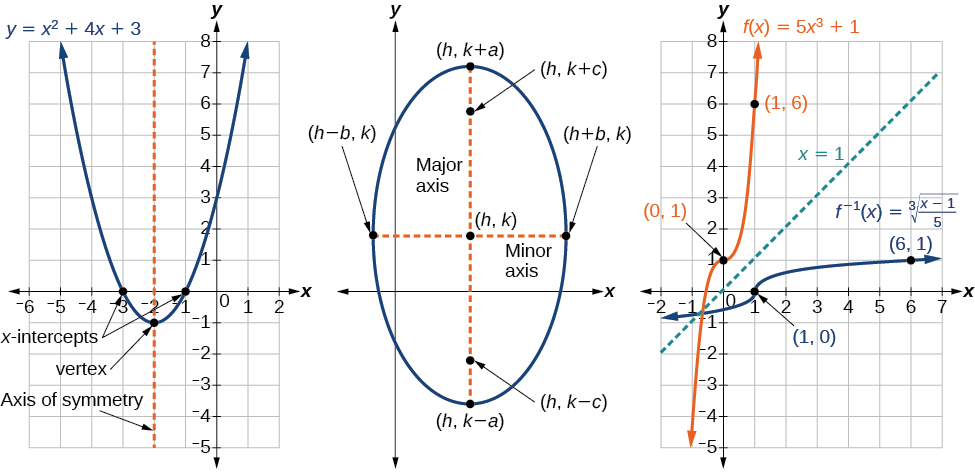# Preface  (Page 3/4)

 Page 3 / 4

## Examples

Each learning objective is supported by one or more worked examples, that demonstrate the problem-solving approaches that students must master. The multiple Examples model different approaches to the same type of problem, or introduce similar problems of increasing complexity.

All Examples follow a simple two- or three-part format. The question clearly lays out a mathematical problem to solve. The Solution walks through the steps, usually providing context for the approach—in other words, why the instructor is solving the problem in a specific manner. Finally, the Analysis (for select Examples) reflects on the broader implications of the Solution just shown. Examples are followed by a Try It question, as explained below.

## Figures

Algebra and Trigonometry contains figures and illustrations, the vast majority of which are graphs and diagrams. Art throughout the text adheres to a clear, understated style, drawing the eye to the most important information in each figure while minimizing visual distractions. Color contrast is employed with discretion to distinguish between the different functions or features of a graph.## Supporting features

Four unobtrusive but important features contribute to and check understanding.

•A “How To” is a list of steps necessary to solve a certain type of problem. A How To typically precedes an Example that proceeds to demonstrate the steps in action.
•A “Try It” exercise immediately follows an Example or a set of related Examples, providing the student with an immediate opportunity to solve a similar problem. In the Web View version of the text, students can click an Answer link directly below the question to check their understanding. In the PDF, answers to the Try-It exercises are located in the Answer Key.
•A Q&A may appear at any point in the narrative, but most often follows an Example. This feature pre-empts misconceptions by posing a commonly asked yes/no question, followed by a detailed answer and explanation.
•The “Media” icon appears at the conclusion of each section, just prior to the Section Exercises. This icon marks a list of links to online video tutorials that reinforce the concepts and skills introduced in the section.

While we have selected tutorials that closely align to our learning objectives, we did not produce these tutorials, nor were they specifically produced or tailored to accompany Algebra and Trigonometry .

## Section exercises

Each section of every chapter concludes with a well-rounded set of exercises that can be assigned as homework or used selectively for guided practice. With over 6300 exercises across the 13 chapters, instructors should have plenty from which to choose 6,367 total exercises. Includes Chapter Reviews and Practice Tests. .

Section Exercises are organized by question type, and generally appear in the following order:

• Verbal questions assess conceptual understanding of key terms and concepts.
• Algebraic problems require students to apply algebraic manipulations demonstrated in the section.
• Graphical problems assess students’ ability to interpret or produce a graph.
• Numeric problems require the student to perform calculations or computations.
• Technology problems encourage exploration through use of a graphing utility, either to visualize or verify algebraic results or to solve problems via an alternative to the methods demonstrated in the section.
• Extensions pose problems more challenging than the Examples demonstrated in the section. They require students to synthesize multiple learning objectives or apply critical thinking to solve complex problems.
• Real-World Applications present realistic problem scenarios from fields such as physics, geology, biology, finance, and the social sciences.

#### Questions & Answers

x exposant 4 + 4 x exposant 3 + 8 exposant 2 + 4 x + 1 = 0
x exposent4+4x exposent3+8x exposent2+4x+1=0
HERVE
How can I solve for a domain and a codomains in a given function?
ranges
EDWIN
Thank you I mean range sir.
Oliver
proof for set theory
don't you know?
Inkoom
find to nearest one decimal place of centimeter the length of an arc of circle of radius length 12.5cm and subtending of centeral angle 1.6rad
factoring polynomial
find general solution of the Tanx=-1/root3,secx=2/root3
find general solution of the following equation
Nani
the value of 2 sin square 60 Cos 60
0.75
Lynne
0.75
Inkoom
when can I use sin, cos tan in a giving question
depending on the question
Nicholas
I am a carpenter and I have to cut and assemble a conventional roof line for a new home. The dimensions are: width 30'6" length 40'6". I want a 6 and 12 pitch. The roof is a full hip construction. Give me the L,W and height of rafters for the hip, hip jacks also the length of common jacks.
John
I want to learn the calculations
where can I get indices
I need matrices
Nasasira
hi
Raihany
Hi
Solomon
need help
Raihany
maybe provide us videos
Nasasira
Raihany
Hello
Cromwell
a
Amie
What do you mean by a
Cromwell
nothing. I accidentally press it
Amie
you guys know any app with matrices?
Khay
Ok
Cromwell
Solve the x? x=18+(24-3)=72
x-39=72 x=111
Suraj
Solve the formula for the indicated variable P=b+4a+2c, for b
Need help with this question please
b=-4ac-2c+P
Denisse
b=p-4a-2c
Suddhen
b= p - 4a - 2c
Snr
p=2(2a+C)+b
Suraj
b=p-2(2a+c)
Tapiwa
P=4a+b+2C
COLEMAN
b=P-4a-2c
COLEMAN
like Deadra, show me the step by step order of operation to alive for b
John
A laser rangefinder is locked on a comet approaching Earth. The distance g(x), in kilometers, of the comet after x days, for x in the interval 0 to 30 days, is given by g(x)=250,000csc(π30x). Graph g(x) on the interval [0, 35]. Evaluate g(5)  and interpret the information. What is the minimum distance between the comet and Earth? When does this occur? To which constant in the equation does this correspond? Find and discuss the meaning of any vertical asymptotes.
The sequence is {1,-1,1-1.....} hasByByByByBy David Martin